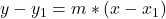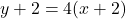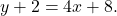## What is the question of the line that passes through the point (-2,-2) and has a slope of 4. Plz someone answer

Question

What is the question of the line that passes through the point (-2,-2) and has a slope of 4. Plz someone answer

in progress 0
2 months 2021-08-22T18:19:48+00:00 1 Answers 1 views 0

We have the information that the slope is 4 and the line goes through the point (-2,-2). With this information, we can make a linear equation in a point slope form (, so the equation would be, or simplified,in order to solve for y (to make it a slope-intercept equation), we must subtract 2 from both sides. This gives us the equation y=4x+6. Hope this helps!